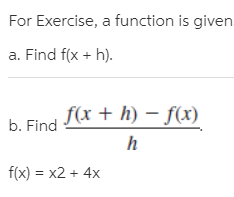# For Exercise, a function is given a. Find f(x + h). b. Find I* + h) – f(x) f(x) = x2 + 4x

Questionhelp_outlineImage TranscriptioncloseFor Exercise, a function is given a. Find f(x + h). b. Find I* + h) – f(x) f(x) = x2 + 4x fullscreen